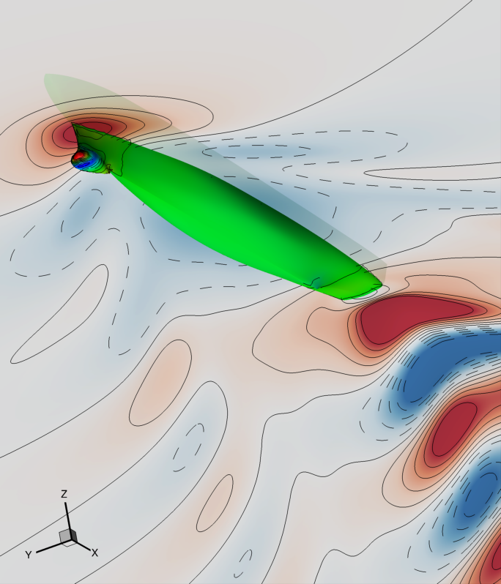# L2-Sea model¶

## Overview¶

The model provides the calm-water total resistance of a destroyer-type vessel as a function of the advancing speed (Froude number) and up to 14 design variables for the shape modification. The parent vessel under investigation is the DTMB 5415, an hull-form widley used for towing tank experiments, computational fluid dynamics studies, and shape optimization. The model is available as an open-source fortran code for the solution of potential flow equations at CNR-INM, MAO Research Group repository.## Run¶

docker run -it -p 4242:4242 linusseelinger/model-l2-sea


## Properties¶

Model

Description

forward

l2-sea

### forward¶

Mapping

Dimensions

Description

input



The first input is the Froude number (from 0.25 to 0.41); the second is the draft (from -6.776 to -5.544); the other 14 are the $$x$$ design variables for the shape modification with $$-1\leq x_i \leq 1$$ for $$i=1,\dots,14$$.

output



The first output is the model scale total resistance ($$R_\mathrm{T}$$) in Newton, whereas the other four are geometrical constraints (negative to be satisfied), related to the beam, draft, and sonar dome dimensions.

Feature

Supported

Evaluate

True

False

ApplyJacobian

False

ApplyHessian

False

Config

Type

Default

Description

fidelity

integer

1

Fidelity level for the total resistance evaluation associated to the numerical grid discretization. Fidelity goes from 1 to 7, where 1 is highest-fidelity level (finest grid) and 7 is the lowest-fidelity level (coarsest grid).

sinkoff

character

‘y’

Enabling hydrodynamics coupling with the rigid-body equation of motions for the ship sinkage. ‘n’ enables, ‘y’ disables.

trimoff

character

‘y’

Enabling hydrodynamics coupling with the rigid-body equation of motions for the ship trim. ‘n’ enables, ‘y’ disables.

## Mount directories¶

Mount directory

Purpose

/output

\texttt{ASCII} files for visualization of pressure distribution along the hull \texttt{pre\textit{XXXX}.plt} and free-surface \texttt{intfr\textit{XXXX}.plt} formatted for Tecplot and Paraview, where \texttt{\textit{XXXX}} is the Froude number.

## Source code¶

Model sources here.

## Description¶

This model describes the calm-water resistance of a destroyer-type vessel by potential flow. Specifically, the vessel under investigation is the DTMB 5415 (at model scale), which is a widely used benchmark for towing tank experiments, CFD studies, and hull-form optimization, considering both deterministic and stochastic formulations.

Potential flow solver is used to evaluate the hydrodynamic loads, based on the Laplacian equation

(1)$\begin{equation} \nabla^2\phi = 0 \end{equation}$

where $$\phi$$ is the velocity scalar potential, satisfying $$\mathbf{u}=\nabla\phi$$ and $$\mathbf{u}$$ is the flow velocity vector. The velocity potential $$\phi$$ is evaluated numerically through the Dawson linearization of the potential flow equations, using the boundary element method. Finally, the total resistance is estimated as the sum of the wave and the frictional resistance: the wave resistance component is estimated by integrating the pressure distribution over the hull surface, obtained using the Bernoulli theorem

(2)$\begin{equation} \frac{p}{\rho} + \frac{\left(\nabla\phi\right)^2}{2}-gz = cost; \end{equation}$

the frictional resistance component is estimated using a flat-plate approximation based on the local Reynolds number.

The steady 2 degrees of freedom (sinkage and trim) equilibrium is achieved considering iteratively the coupling between the hydrodynamic loads and the rigid-body equation of motion.

The model can exploit multiple grid discretization levels, whose details can be found in Pellegrini et al. (2022).